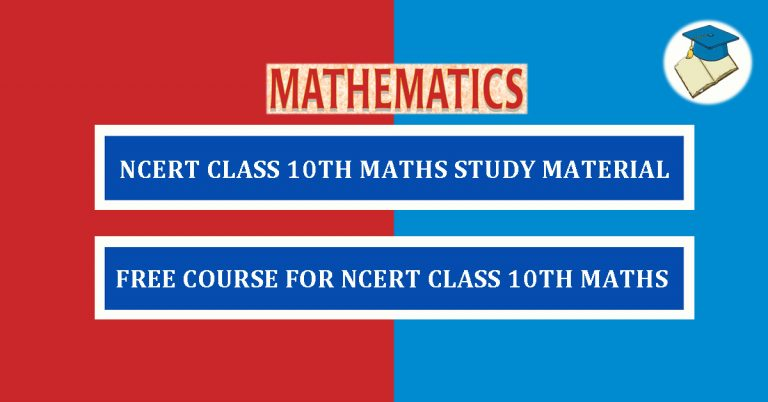# NCERT Class 10th Maths Study Material## Welcome to the NCERT Class 10th Maths Study Material and Solutions

Welcome students to this free NCERT Class 10th Maths study material and solutions. In this course, we will provide you free chapter-wise study material for NCERT Class 10th Mathematics. We will also provide you solutions for exercises. The study material consists of articles, PDFs and videos.

### NCERT Class 10th Maths Chapter-wise Study Material

The NCERT Class 10th syllabus is divided into 15 chapters. These 15 chapters are:

1. Real Numbers
2. Polynomials
3. Pair of Linear Equations in Two Variables
5. Arithmetic Progressions
6. Triangles
7. Coordinate Geometry
8. Introduction to Trigonometry
9. Some Applications of Trigonometry
10. Circles
11. Constructions
12. Areas Related to Circles
13. Surface Areas and Volumes
14. Statistics
15. Probability

Lets’ start chapter-wise study of Class 10th Maths.